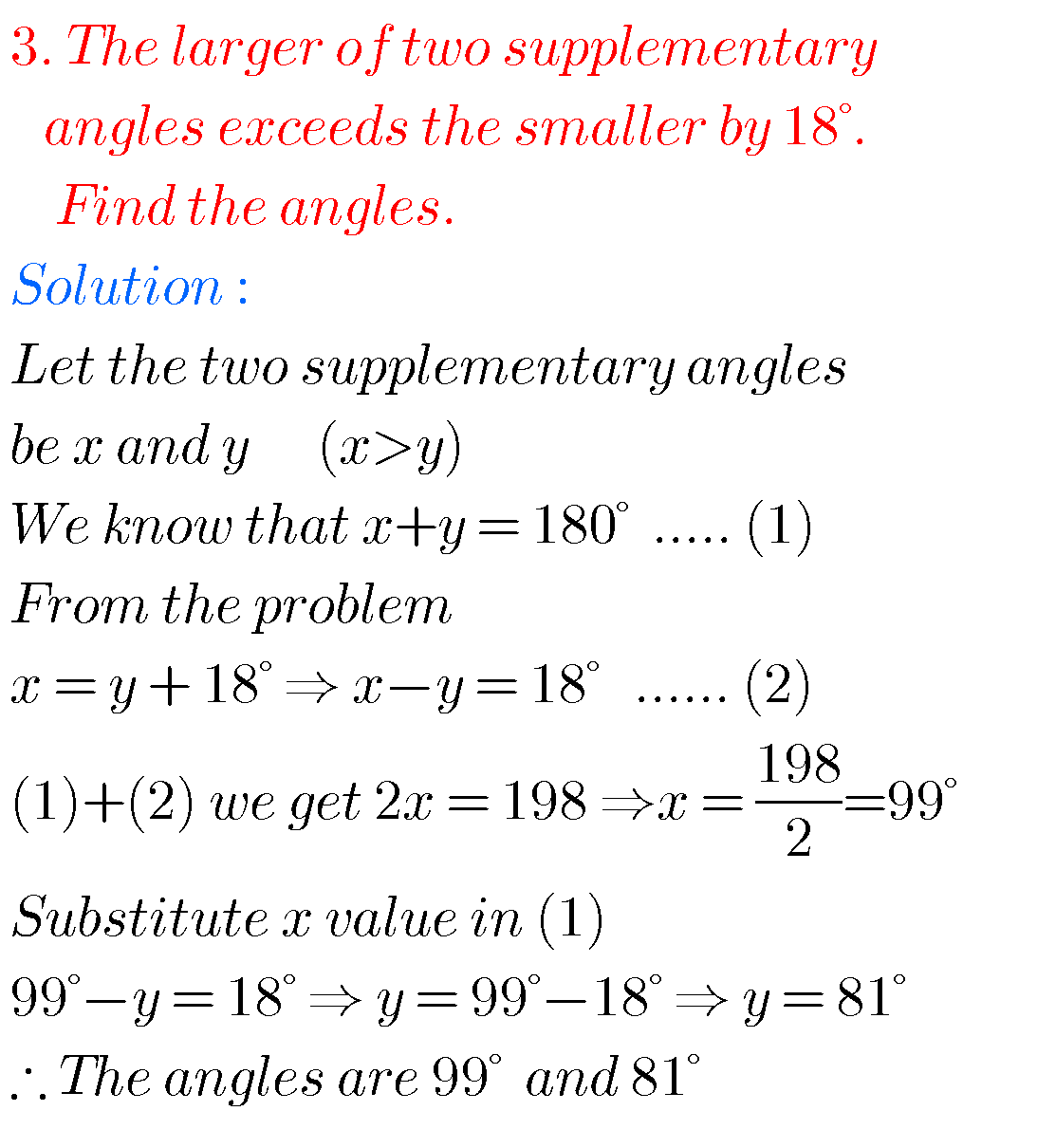## SSC maths solutions for Pair of Linear Equations in Two Variables class 10

SSC maths solutions for Pair of Linear Equations in Two Variables SSC mathematics class 10 Pair of Linear Equations in Two Variables solutions for text book exercises. These solutions are very easy to understand. First study the text book well. Exercise 4.1, exercise 4.2 and exercise 4.3  solutions are given. Observe the given solutions and …